# Normalisation vs. Standardisation

## Introduction

Visualization is the most effective approach to understand the working of data. Instead of studying data in the form of a table, it is easier to visualize and comprehend rapidly. Various exciting insights can be gained from our data by understanding the distribution of the data. The two primary ways to do so are Normalisation and Standardisation. We will be discussing the difference between the two.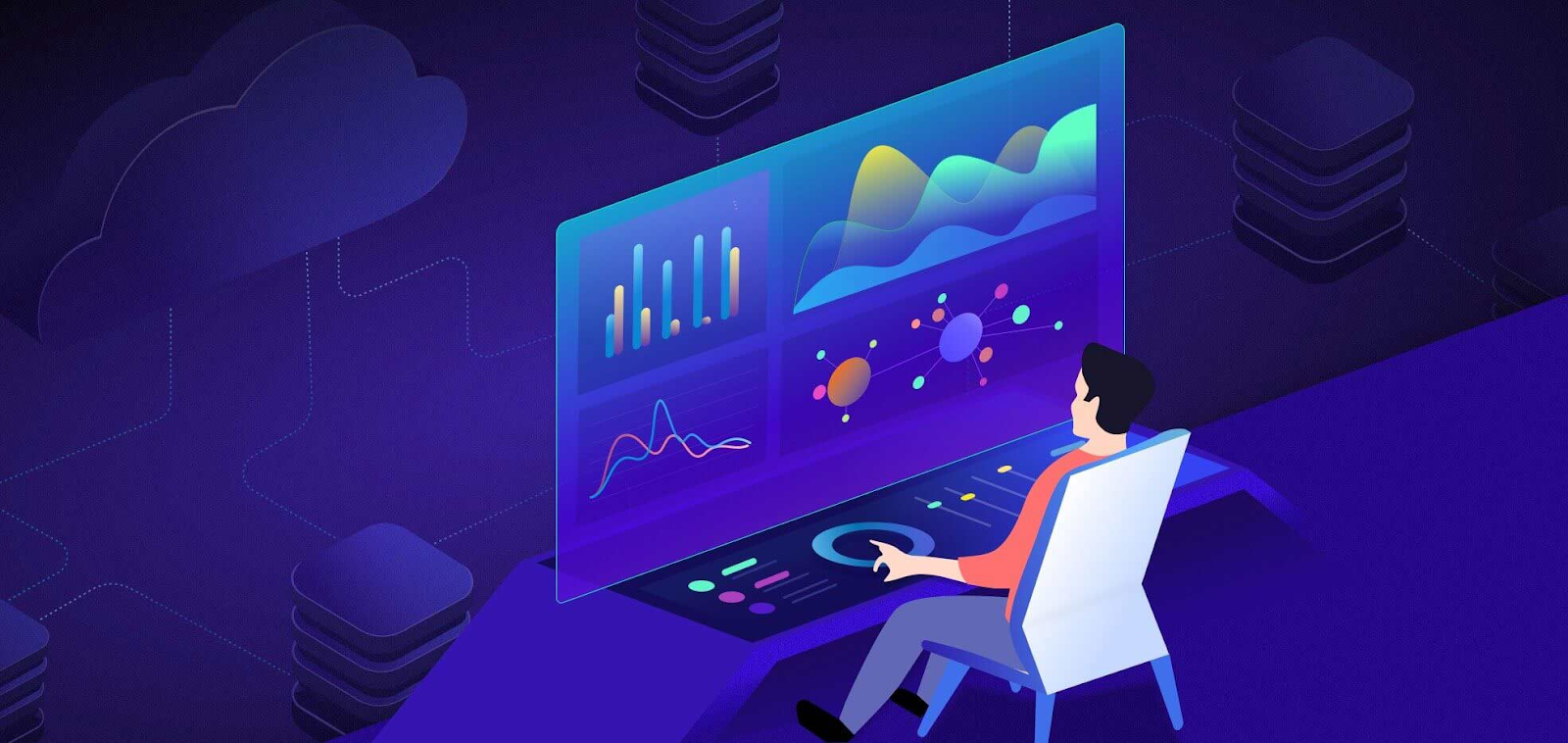## What is Normalization?

The process of arranging the data in a database is known as Normalization. It is a scaling technique used to reduce redundancy in which the values are shifted and scaled in a range of 0 and 1. Normalization is used to remove the unwanted characteristics from the dataset, and it is useful when there are no outliers as it can not handle them. Mathematically, Normalisation is denoted as: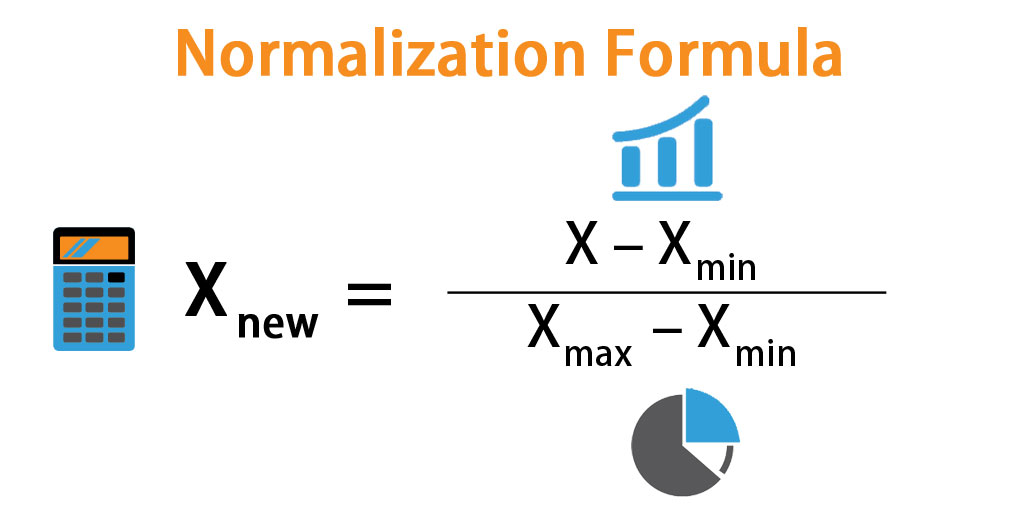## What is Standardisation?

Data standardization is a process in which the data is restructured in a uniform format. In statistics, standardization compares the variables by putting all the variables on the same scale. It is done by transforming the features by subtracting from the mean and dividing by the standard deviation. This process is also known as the Z-score. Mathematically, Standardisation is denoted as: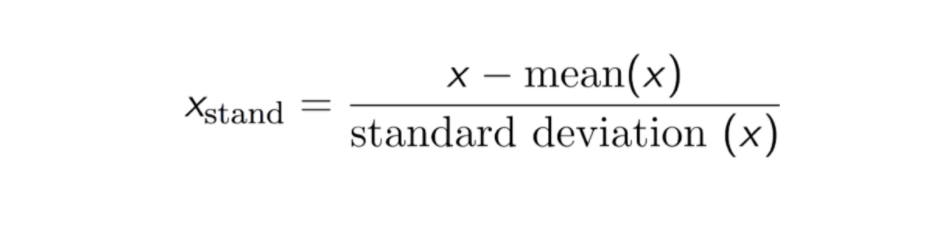## Normalisation vs. Standardisation using sklearn library

### Implementing Normalisation

``````#importing the Normalizer from sklearn
from sklearn.preprocessing import Normalizer
#Creating a sample data array
X = [[4, 1, 2, 2],[1, 3, 9, 3],[5, 7, 5, 1]]
transformer = Normalizer().fit(X)  # fit does nothing.
transformer
Normalizer()
transformer.transform(X)``````

Output: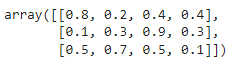### Implementing Standardisation

``````#importing the StandardScaler from sklearn
from sklearn.preprocessing import StandardScaler
#Creating a sample data array
data = [[0, 0], [0, 0], [1, 1], [1, 1]]
scaler = StandardScaler()
print(scaler.fit(data))
print(scaler.mean_)
print(scaler.transform(data))
print(scaler.transform([[2, 2]]))``````

Output: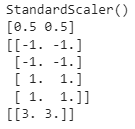In Normalisation, the change in values is that they are at a standard scale without distorting the differences in the values. Whereas, Standardisation assumes that the dataset is in Gaussian distribution and measures the variable at different scales, making all the variables equally contribute to the analysis.

## Normalisation vs. Standardisation

Normalisation vs. standardisation is an ultimate doubt among machine learning beginners.

Normalisation is suitable to use when the data does not follow Gaussian Distribution principles. It can be used in algorithms that do not assume data distribution, such as K-Nearest Neighbors and Neural Networks.

On the other hand, standardisation is beneficial in cases where the dataset follows the Gaussian distribution. Unlike Normalization, Standardisation is not affected by the outliers in the dataset as it does not have any bounding range.

Applying Normalization or Standardisation depends on the problem and the machine learning algorithm. There are no definite rules as to when to use Normalization or Standardisation. One can fit the normalized or standardized dataset into the model and compare the two.

It is always advisable to first fit the scaler on the training data and then transform the testing data. This would prohibit data leakage during the model testing process, and the scaling of target values is generally not required.

Q1. Should we normalize or standardize images?

Ans. If the image dataset is too small, then Standardisation is preferred because if we apply Normalisation, the dataset values will compress get decrease.

Q2. Does Normalisation changes the standard deviation?

Ans. Normalization reduces the values of standard deviation as the values are compressed; hence standard deviation is also shortened.

Q3. Does Normalisation affect variance?

Ans. After normalizing, the variance is decreased massively.

## Key Takeaways

This article summarised the significant differences between normalisai\tiona and Standardisation, including the sklearn implementation of both. If you’re interested in going deeper, Check out our industry-oriented machine learning course curated by our faculty from Stanford University and Industry experts.